Next: Example 10.3: The RL Up: Inductance Previous: Example 10.1: Mutual induction

## Example 10.2: Energy density of electric and magnetic fields

Question: In a certain region of space, the magnetic field has a value of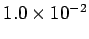T, and the electric field has a value of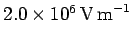. What is the combined energy density of the electric and magnetic fields?

Answer: For the electric field, the energy density is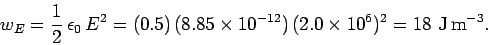For the magnetic field, the energy density is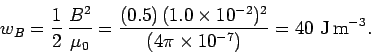The net energy density is the sum of the energy density due to the electric field and the energy density due to the magnetic field: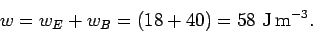Richard Fitzpatrick 2007-07-14